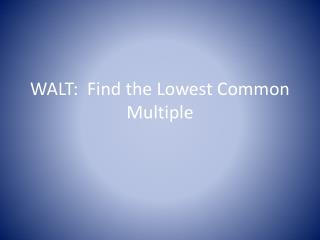DownloadDownload PresentationWALT: Find the Lowest Common Multiple

WALT: Find the Lowest Common Multiple

Télécharger la présentationWALT: Find the Lowest Common Multiple

- - - - - - - - - - - - - - - - - - - - - - - - - - - E N D - - - - - - - - - - - - - - - - - - - - - - - - - - -
Presentation Transcript

1. WALT: Find the Lowest Common Multiple

2. What are the multiples of: • 5 • 10 • 7 • 18

3. How do we know if a number is a multiple of: • 2 • 4 • 5 • 10 • 100

4. Are the following numbers multiples of 2? • 246 • 1067 • 20000 • Three million

5. Are the following numbers multiples of 4? • 300 • 235 • 410 • Five hundred and thirty two

6. Are the following numbers multiples of 5? • 1000 • 25456 • 5555 • 1025 • Three million two hundred and fifty six

7. Which of the numbers below are multiples of both 2 AND 5? 20 40 45 90 76 105 200 305 40 42Give three numbers which are multiples of 2 and 5. What can you say about numbers which are multiples of 2 and 5?

8. What is a Lowest Common Multiple? • Consider the numbers 2 and 5 • The lowest common multiple is the smallest number which is the multiple of both. • Multiples of 2 are: 2, 4, 6, 8, 10, 12, 14, … • Multiples of 5 are: 5, 10, 15, 20, 25, … • Which is the smallest number that occurs in BOTH lists? • This is the LOWEST COMMON MULITPLE.

9. What is the LOWEST COMMON MULTIPLE of: • 2 and 7 • 3 and 7 • 5 and 3 • 5 and 7

10. Question: • Decide with your partner the answer to the question below. You must EXPLAIN your answer and give examples. • Is the lowest common multiple of two numbers always found by multiplying those two numbers together? • E.g LCM of 2 and 7 is 2 x 7 = 14

11. Find the LCM of: • 4 and 40 • 4 and 8 • 4 and 6 • 12 and 4

12. Design a poster • Make a poster explaining what the Lowest Common Multiple of a pair of number is. • Make sure you give some examples • Give a method for finding the LCM.